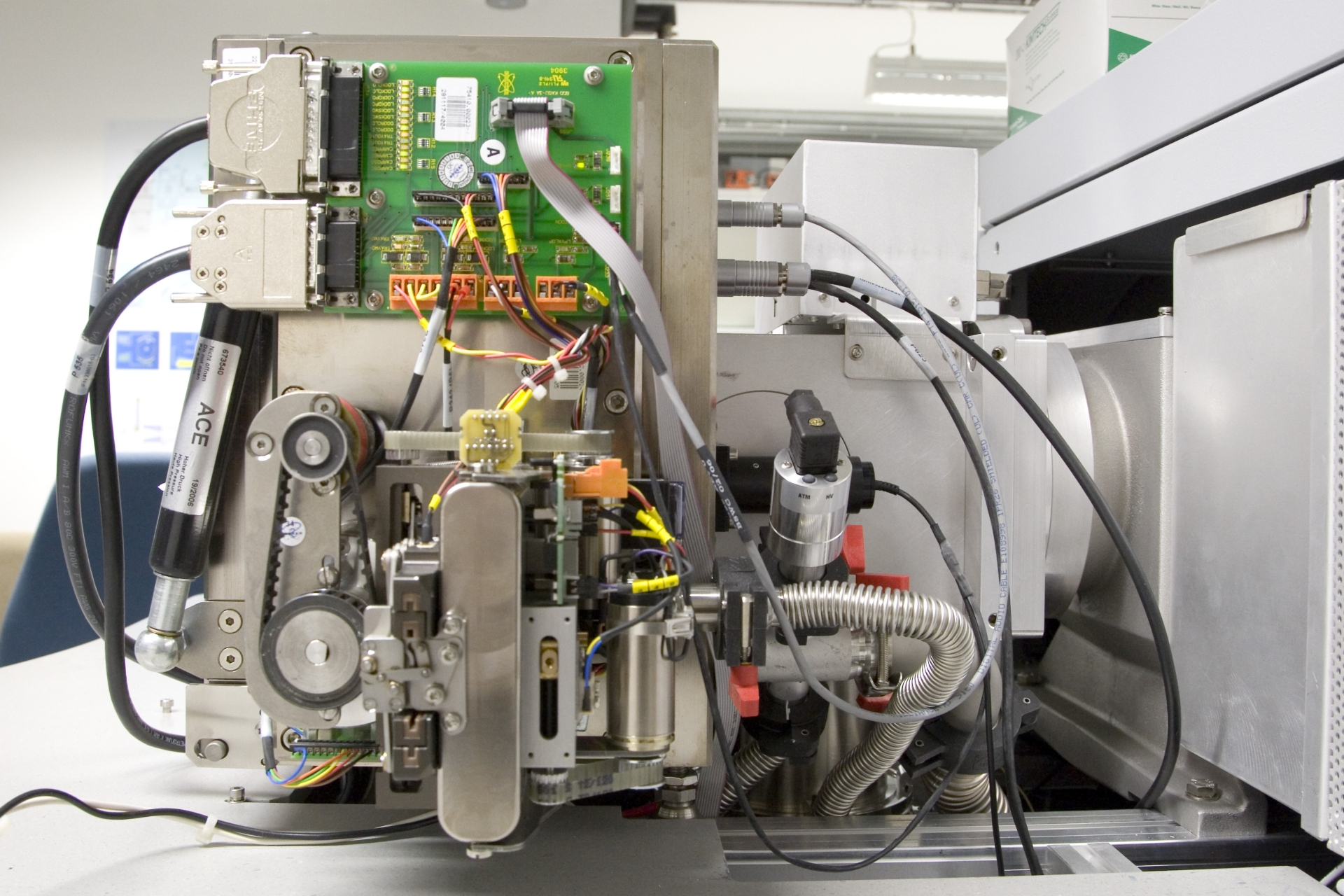# Mass Scale

Mass spectrometers do not directly measure mass, but the mass-to-charge ratio of ions. Strictly speaking, in physics we use ion mass in kilograms [kg] and the electric charge in Coulomb [C].  As we will see in a moment, using a scale in units of kg C–1 would be very unpractical.

Let us simply try to apply the kg C–1 scale to a molecule of ethanol, MW = 0.046 kg mol–1. The mass of the molecule is obtained by dividing the molecular weight by Avogadro’s constant (6.022 ´ 1023). Thus, for a molecule of ethanol, we calculate a mass of 7.64 ´ 10–26 kg. A singly charged molecular ion carries one electron less than the corresponding neutral molecule, and therefore, it carries exactly one elementary charge, i.e., 1.602 ´ 10–19 C. The molecular ion would thus be detected at 4.77 ´ 10–7 kg C–1. Who is really going to like such numbers to work with?

In mass spectrometry, the mass-to-charge ratio, m/z, is defined as a dimensionless quantity that can best be understood as the ratio of the dimensionless mass number divided by the dimensionless number of elementary charges of the ion. As long as singly charged ions are concerned, the numerical value of m/z equals the numerical value of the ionic mass on the atomic mass scale. For example, the molecular ion of ethanol, 46 u, is detected at m/z 46.